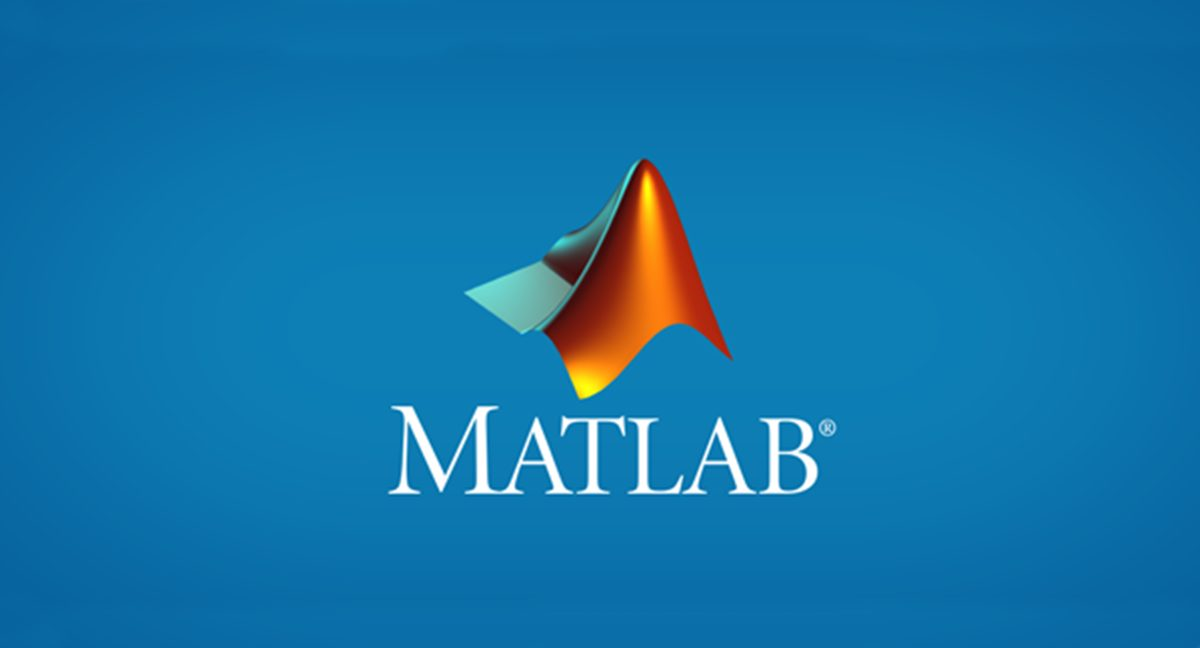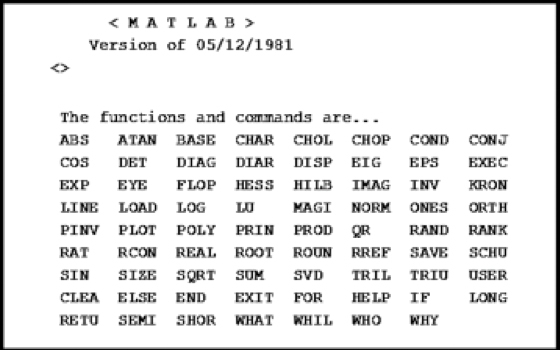## Introduction :

In this session, we want to answer three questions: What is MATLAB? Why learn MATLAB? How to learn MATLAB? In short, in this session, our goal is to introduce you to MATLAB software and its capabilities.

## What is MATLAB?Figure 1: MATLAB software logo

AT MATLAB is a programming platform  designed for engineers and scientists. The heart of the MATLAB platform is the MATLAB programming language. A matrix-based language that allows you to perform mathematical and computational operations in the simplest possible way. MATLAB stands for ” MAT rix LAB Ratory” means “matrix laboratory” is. From the name of MATLAB software, it is clear that you are dealing with software based on a matrix. Naturally, it can also be assumed that this is a software for doing math. Also, the MATLAB software logo (Figure 1) indicates that you are dealing with engineering software.

 In the paragraph above, we said that MATLAB is a programming platform. We mean that MATLAB itself is a comprehensive and complete software. Many programming languages ​​do not have their own environment or software.## History of MATLAB

Cleve Moler, head of the Department of Computer Science at the University of New Mexico, began building and developing MATLAB in the late 1970s. He designed the software for his students. Students needed math and algebraic calculations and had to work with Fortran problem language. With MATLAB, you no longer needed to learn Fortran’s difficult language to do algebra. MATLAB software spread rapidly to other universities. And because of his power in linear algebra and mathematics, he became very popular in the applied mathematics community. Jack Little became acquainted with MATLAB software during a meeting with Moler at Stanford University in 1983. He saw MATLAB as software with commercial potential. He joined Moler and Steve Bangert to build the commercial MATLAB. They rewrote MATLAB in C and in 1984 MathWorks Established to continue developing MATLAB software. In 2000, MATLAB was rewritten to a new set of libraries (libraries  LAPACK) for Jbrkhty and work with the matrix.

MATLAB was first developed by researchers and control engineers. But it spread rapidly to many other fields, such as mechanical engineering, civil engineering, computers, mathematics, and so on. This software is even used to teach algebra. Little can be said about the impact of MATLAB in the world of science.

 The LAPACK library stands for Linear Algebra PACKage, which is for linear algebra work. This library was developed by Intel. Today, they have been developed for engineering libraries and software based on Intel algebraic libraries.After what is MATLAB, we want to answer the question of why MATLAB. The examples we have given are readable. We suggest you do not miss…

Why learn MATLAB?

Be careful, MATLAB is not suitable for a programmer. This software is designed for engineers and scientists. We have provided an example for you to make things clearer for you. Imagine you have a mobile app design and development team. Our Academy has asked you to design a mobile app for it. Our Academy has said the general work and left the details of the app to your team. I definitely do not want the academy I want you to come six months later and say that the app is ready, give us the money to go! A professional team first builds a prototype. what does it mean?

That is, it creates a prototype, incomplete, and fast that the employer first approves and then begins the main work. If the employer tells you to change these parts, because it is a prototype, they change easily. They do not worry about wasting time and energy. Once the client approves, the original design work begins. Now how to prototype in mobile app design? Take a look at the image below. Many papers and magic use colour to build a prototype. Of course, today there are software for prototyping mobile apps and sites that we will not discuss. However, with this, the employer sees everything very simply and the manager of the app development team explains it.Of course, there is still an example. For example, large car factories do not suddenly decide to build a car with a new body to build a new car. First, they design the body with software and then go and build a prototype. They do their experiments. Until they finally reach the main production stage. There are many examples of the importance of prototypes. Let’s go to the main point…

The same is true for an engineer and scientist who deals with mathematics. He wants to implement his algorithm or idea as simply as possible. Maybe even writing on paper is easier for him than writing MATLAB! But why is MATLAB commonly used? Because MATLAB is like a calculator for an engineer and a scientist. Instead of trying to solve all the problems on paper, it is time-consuming. He writes it in MATLAB and naturally his job becomes easier. So writing a program and an idea in MATLAB becomes the prototype! Professional programmers can now implement this prototype in a language like C ++ to turn it into a commercial product.

We consider this explanation necessary because in the past we had challenges with different people, why do you implement your project in MATLAB and then go and implement it in C ++ or Java? Well, write in C ++ or Java from the beginning! We hope you know the answer now. We want to implement our idea as soon as possible.MATLAB-NET-JAVA-cpp

Well, it’s okay to take it! What happens? Again, the answer is, do we know if this idea will lead to an answer? For example, for one task we may have five ideas and the fourth idea will be answered. Isn’t it better to determine the success or failure of an idea as soon as possible? So that if we fail, we do not regret the lost time and be happy that we understood soon.

## MATLAB features for engineers and scientists

In this section, we want to show you the features of MATLAB to see why learning MATLAB is valuable. Here are six reasons why MATLAB is suitable for engineers and scientists.

MATLAB is a mathematical language: Engineers and scientists need a programming language that can easily perform mathematical operations. Linear algebra can be easily used in MATLAB. Of course, this simplicity also applies to the implementation of ideas in many areas, such as data analysis, signal and image processing, and control design. That’s why more than 1,500 reference textbooks, such as Gonzalez’s image processing textbook, teach the use of MATLAB.

MATLAB software for engineers and scientists: Everything in MATLAB is designed for engineers and scientists. The names of the functions in MATLAB have been chosen in such a way that they easily remain in people’s minds. The names of functions in MATLAB are very close to their names in mathematics. MATLAB is designed for engineers and scientists, not programmers… So if programmers do not know MATLAB, there is no reason why MATLAB is bad. Rather, it is software for engineers and scientists.

MATLAB Toolbox: MATLAB includes a large set of MATLAB Toolbox. Each toolbox has its own application. For example, if you are a civil, electrical and mechanical engineer, you can use MATLAB’s proprietary civil, electrical and computer toolkit. MATLAB toolkit is very large and includes many disciplines. (Click here to get acquainted with MATLAB toolbox.)
MATLAB is fast: the software is implemented with advanced techniques. This feature makes it possible to perform calculations based on matrices quickly in mathematics.

MATLAB is safe: MATLAB is a paid software. A large team behind MATLAB is constantly working. This makes MATLAB much more reliable than open-source software.

Speed ​​of implementation in MATLAB: As an engineer or scientist, you can easily implement any idea in your work in MATLAB. Imagine trying to implement your idea in Java. You definitely have to spend a lot more time in Java.

## How to learn MATLAB?

Our Free MATLAB training course has been prepared with the aim of teaching MATLAB to anyone who has no familiarity with MATLAB or programming. So how do we learn MATLAB? Our answer is, just follow the free MATLAB tutorial. Read each session carefully and practice arithmetic.

.